# Catalan numbers

The Catalan numbers (1, 2, 5, 14, 42, 132, 429, 1430, 4862, 16796, 58786, 208012, 742900, 2674440, 9694845, ...), named after Eugène Charles Catalan (1814--1894), arise in a number of problems in combinatorics. They can be computed using this formula: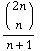Polygon diagrams (code hidden for your protection):

4 sides, 2 ways: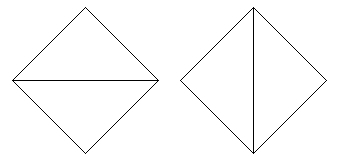5 sides, 5 ways: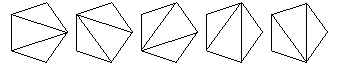6 sides, 14 ways: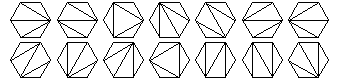7 sides, 42 ways: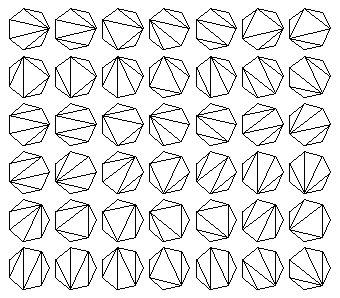8 sides, 132 ways: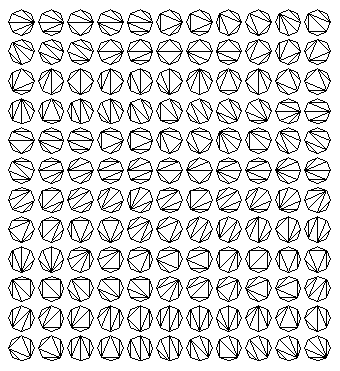9 sides, 429 ways:
(Hidden in file catalan9.gif; around 29K.)

3 numbers:

`(1 (2 3))   ((1 2) 3)`
4 numbers:
```(1 (2 (3 4)))   (1 ((2 3) 4))
((1 2) (3 4))   ((1 (2 3)) 4)
(((1 2) 3) 4)```
5 numbers:
```(1 (2 (3 (4 5))))   (1 (2 ((3 4) 5)))
(1 ((2 3) (4 5)))   (1 ((2 (3 4)) 5))
(1 (((2 3) 4) 5))   ((1 2) (3 (4 5)))
((1 2) ((3 4) 5))   ((1 (2 3)) (4 5))
((1 (2 (3 4))) 5)   ((1 ((2 3) 4)) 5)
(((1 2) 3) (4 5))   (((1 2) (3 4)) 5)
(((1 (2 3)) 4) 5)   ((((1 2) 3) 4) 5)
```
6 numbers:
```(1 (2 (3 (4 (5 6)))))   (1 (2 (3 ((4 5) 6))))
(1 (2 ((3 4) (5 6))))   (1 (2 ((3 (4 5)) 6)))
(1 (2 (((3 4) 5) 6)))   (1 ((2 3) (4 (5 6))))
(1 ((2 3) ((4 5) 6)))   (1 ((2 (3 4)) (5 6)))
(1 ((2 (3 (4 5))) 6))   (1 ((2 ((3 4) 5)) 6))
(1 (((2 3) 4) (5 6)))   (1 (((2 3) (4 5)) 6))
(1 (((2 (3 4)) 5) 6))   (1 ((((2 3) 4) 5) 6))
((1 2) (3 (4 (5 6))))   ((1 2) (3 ((4 5) 6)))
((1 2) ((3 4) (5 6)))   ((1 2) ((3 (4 5)) 6))
((1 2) (((3 4) 5) 6))   ((1 (2 3)) (4 (5 6)))
((1 (2 3)) ((4 5) 6))   ((1 (2 (3 4))) (5 6))
((1 (2 (3 (4 5)))) 6)   ((1 (2 ((3 4) 5))) 6)
((1 ((2 3) 4)) (5 6))   ((1 ((2 3) (4 5))) 6)
((1 ((2 (3 4)) 5)) 6)   ((1 (((2 3) 4) 5)) 6)
(((1 2) 3) (4 (5 6)))   (((1 2) 3) ((4 5) 6))
(((1 2) (3 4)) (5 6))   (((1 2) (3 (4 5))) 6)
(((1 2) ((3 4) 5)) 6)   (((1 (2 3)) 4) (5 6))
(((1 (2 3)) (4 5)) 6)   (((1 (2 (3 4))) 5) 6)
(((1 ((2 3) 4)) 5) 6)   ((((1 2) 3) 4) (5 6))
((((1 2) 3) (4 5)) 6)   ((((1 2) (3 4)) 5) 6)
((((1 (2 3)) 4) 5) 6)   (((((1 2) 3) 4) 5) 6)
```
Tree diagrams:

3 nodes: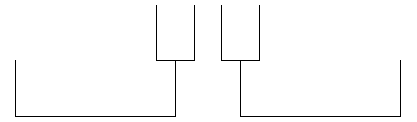4 nodes: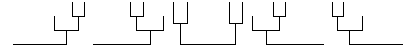5 nodes: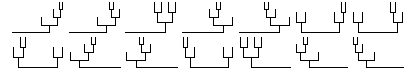6 nodes: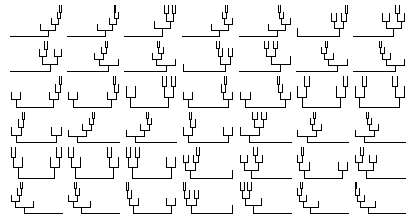7 nodes: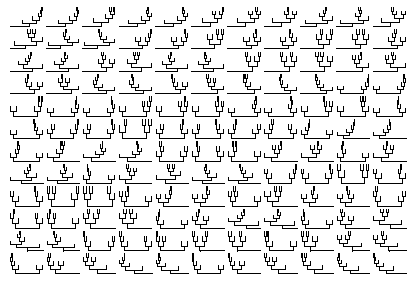8 nodes:
(Tucked away in file cattree8.gif; around 17K.)

2 x 2 grid: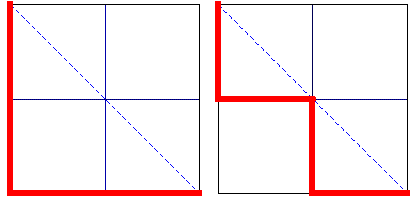3 x 3 grid: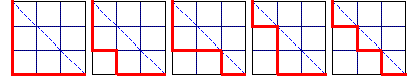4 x 4 grid: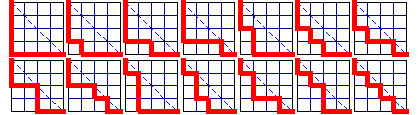5 x 5 grid: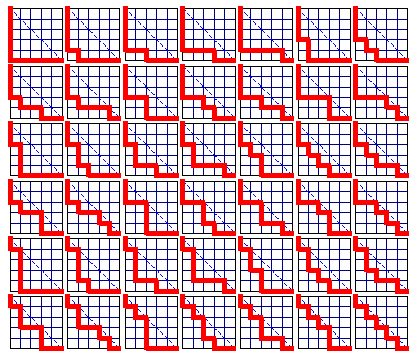6 x 6 grid:
(Out of the way in file catalanpath6.gif; around 24K.)

Designed and rendered using Mathematica 3.0 for the Apple Macintosh. Inspiration and facts (though not figures) by Brian Hayes, "A Question of Numbers", American Scientist, January-February 1996; Steven S. Skiena, Implementing Discrete Mathematics: Combinatorics and Graph Theory with Mathematica, Addison-Wesley, 1990; Fred S. Roberts, Applied Combinatorics, Prentice-Hall, 1984; and D. E. Knuth, Sorting and Searching (vol. 3 of The Art of Computer Programming), Addison-Wesley, 1973. Catalan dates from Florian Cajori, A History of Mathematics, The Macmillan Company, 1922.

See also Martin Gardner, Time Travel and Other Mathematical Bewilderments, chapter 20, W. H. Freeman, 1988; and Ilan Vardi, Computational Recreations in Mathematica, chapter 9, Addison-Wesley, 1991.